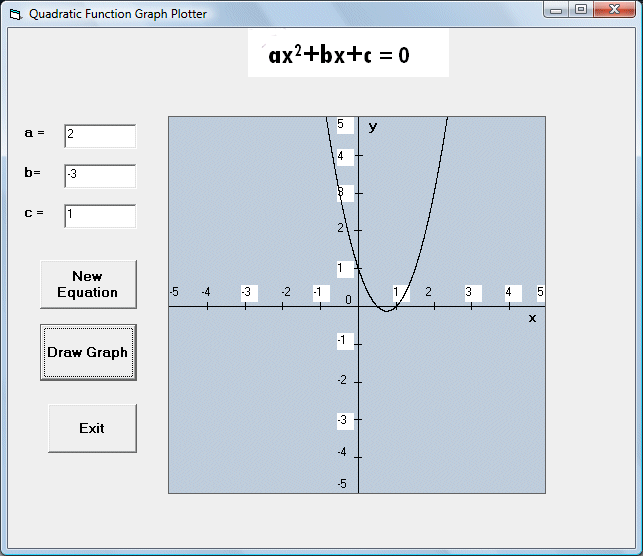This is a program that can plot graphs for quadratic functions. The formula of a quadratic equation is f(x)= ax2+bx+c , where a, b and c are constant.

This program  employs a picture box as the plot area and three text boxes to obtain the values of the coefficients a, b, c of the quadratic equation from the users. We also need to modify the scale factor in the properties windows of the picture box. We are using a scale of 0.5 cm to represent 1 unit .
Besides, we need to make some transformation as the coordinates in VB start from top left but we want it to start from the middle. We can use the Pset method to draw the graph using a very small increment. Pset is a method that draws a dot on the screen, the syntax is

Pset(x,y), color

Where (x,y) is the coordinates of the dot and color is the color of the dot. Using the For Next loop together with Pset, we can draw a line on the screen.

### The Interface### The Code

Private Sub cmd_draw_Click()
Dim a, b, c As Integer
Dim w, v As Single
a = Val(txt_a.Text)
b = Val(txt_b.Text)
c = Val(txt_c.Text)

'Using a scale of 0.5 cm to represent i unit to draw the graph
' Need to make some transformation as the coordinates in VB start from top left

For w = 0 To 10 Step 0.001

v = a * (5 - w) ^ 2 - b * (5 - w) + c
pic_graph.PSet (w, 5 - v)

Next w

End Sub

Private Sub Command1_Click()
pic_graph.Cls

txt_a.Text = ""
txt_b.Text = ""
txt_c.Text = ""

End Sub